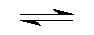## Hexa Aqua Calcium(II+) Cation Complex Hydrolise by Aris Kaksis  Department of medical Biochemistry, Riga Stradin University RSU

At equilibrium, the following equilibrium equation is established withits conjugate base:
Ca2+(H2O)6(aq)      +      H2O(l)Ca2+(H2O)5(OH-)(aq)   + H3O+(aq)
acid                                                                     conjugate base
The acid and its conjugate base have different colours. At low pH valuesthe concentration of H3O+is high and so
the equilibrium position lies to the left. The equilibrium solution has the colour A. At high pH values,the concentration
of H3O+is low - the equilibrium position thus lies to the right and the equilibrium solution has colour B.

blue (Acid) pHhydrolize=12.7             red (Base)

Ca6H2O.skc   Ca6H2O.tgf   CaX6H2O.mol

We can apply equilibrium law to Brønsted equilibria - in generalfor a weak acid calcium complex:
Keq= ( [H3O+]·[Ca2+(H2O)5(OH-)]/ ([Ca2+(H2O)6][H2O])) eq
Keq is known as the Brønsted equilibria constant. The pH for hydrolyse is calculated at this point when:
[Ca2+(H2O)5(OH-)]  =   [Ca2+(H2O)6]
So from equation: Keq = [H3O+]/ [H2O] =10 -12.7/[H2O] = 10 -12.7/55.33 = 10 -14.44
Keq = [H3O+]/  [H2O] ; Keq[H2O]= [H3O+] =10-12.7; -log(Keq[H2O])= 12.7 = pH = -log([H3O+])
The pH of the solution at its equilibrium point is called the pH andis the pH at which half of the calcium
is in its acidform and the other half in the form of its conjugatebase.
At a low pH<12.7, a weak acid aqua complex of calcium ion is almost entirely in the [Ca2+(H2O)6]form, the
concentration of which predominates. As the pH increases pH>12.7- the concentration of [Ca2+(H2O)6] decreases
and the equilibrium is pushed to the right to conjugate base [Ca2+(H2O)5(OH-)]form, the concentration of which predominates.

Aqua complex of calcium

We can apply equilibrium law to complexe stability constant equilibria - in general for a acid form calcium cation:
Ca2+(aq)     +   OH- (aq)Ca2+(OH-)(aq)
blue (Acid)                                                            red (Base)
Kstab=[Ca2+(OH-)]/([Ca2+][OH-])=101.3= ([Ca2+(OH-)][H3O+])/ ([Ca2+][10-14])
Kstab is known as the Complexe stability constant. The pH for hydrolyse is calculated at this point when:
[Ca2+(OH-)]  =   [Ca2+]
So from equation: Kstab = [H3O+]/ 10-14 =10 1.3
Kstab10-14= [H3O+] =10-12.7; -log(Keq10-14) = 12.7= pH = -log([H3O+])
The pH of the solution at its equilibrium point is called the pH and is the pH at which half of the calcium is in
its conjugate base and the other half in the form of its acid form.

Complex maker of calcium cation
At a low pH<12.7, a weak acid aqua complex of calcium ion isalmost entirely in the Ca2+form free cation, the
concentration of which predominates. As the pH increasespH>12.7 - the concentration of [Ca2+]decreases and
the equilibrium is pushed to the right to conjugate base    [Ca2+(OH-)]form, the concentration of which predominates.

Literature

Handbook of  Chemical Equilibriain Analytical Chemistry. S.Kortly, L.Sucha. New York , Ellis HorwoodLTD. 1985. 414.p.